Function Repository Resource:

# ColorGraphEdges

Color the edges of a graph so no edges incident to each other have the same color

Contributed by: Peter Cullen Burbery
 ResourceFunction["ColorGraphEdges"][graph] colors the edges of graph so no edges incident to each other have the same color. ResourceFunction["ColorGraphEdges"][graph, colors] uses the color function colors.

## Details

The vertices are colored using ColorData by default.
Finding edge colorings for graphs is an NP complete problem.

## Examples

### Basic Examples (2)

Color the edges of the Petersen graph:

 In:=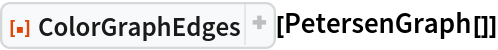Out=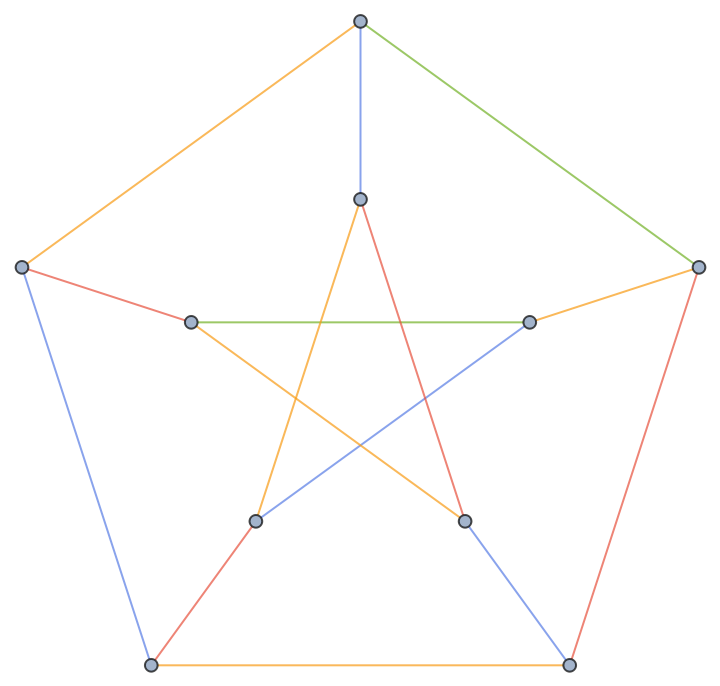Use a different coloring list with ColorData:

 In:=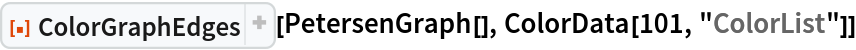Out=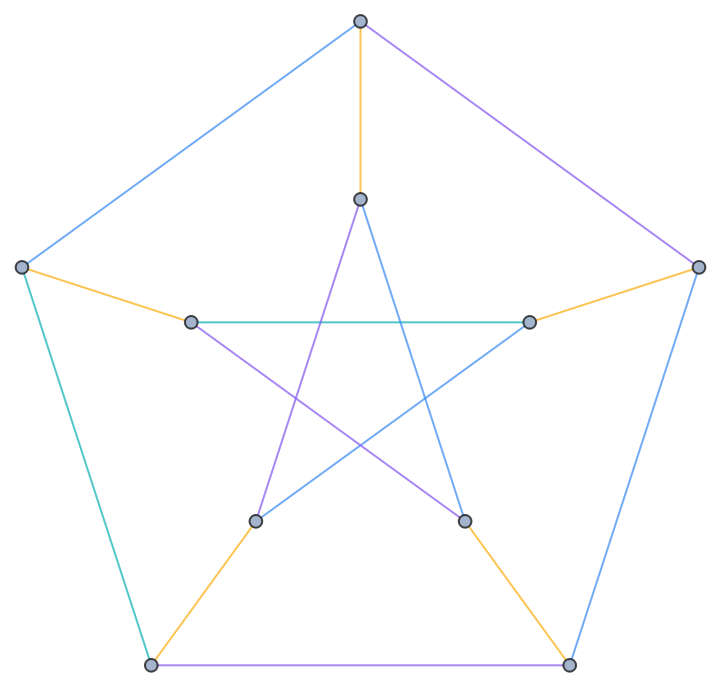### Scope (1)

Color the edges of the skeleton of the Csaszar polyhedron:

 In:=Out=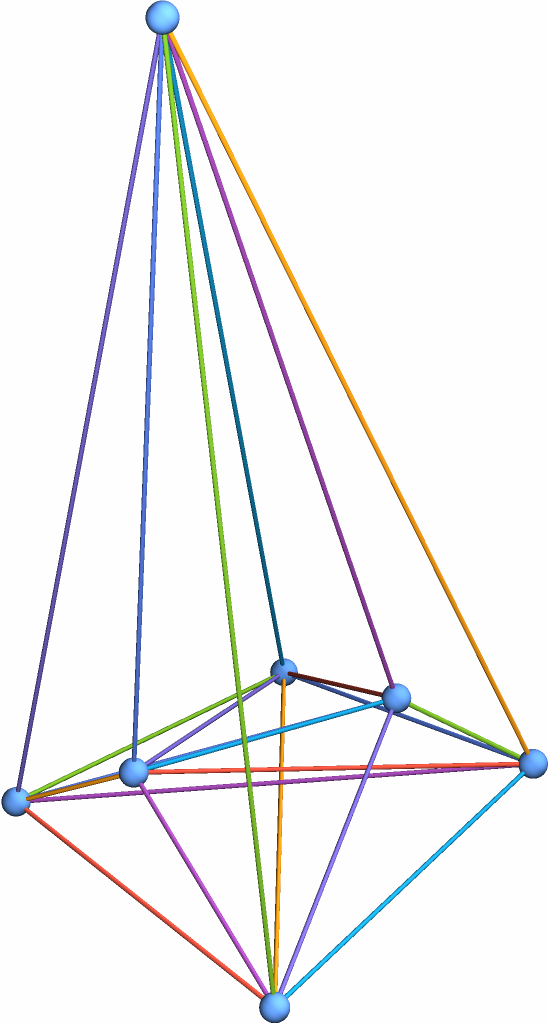### Options (1)

ColorGraphVertices takes the same options as Graph:

 In:=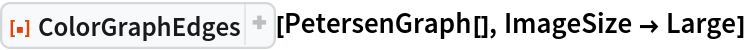Out=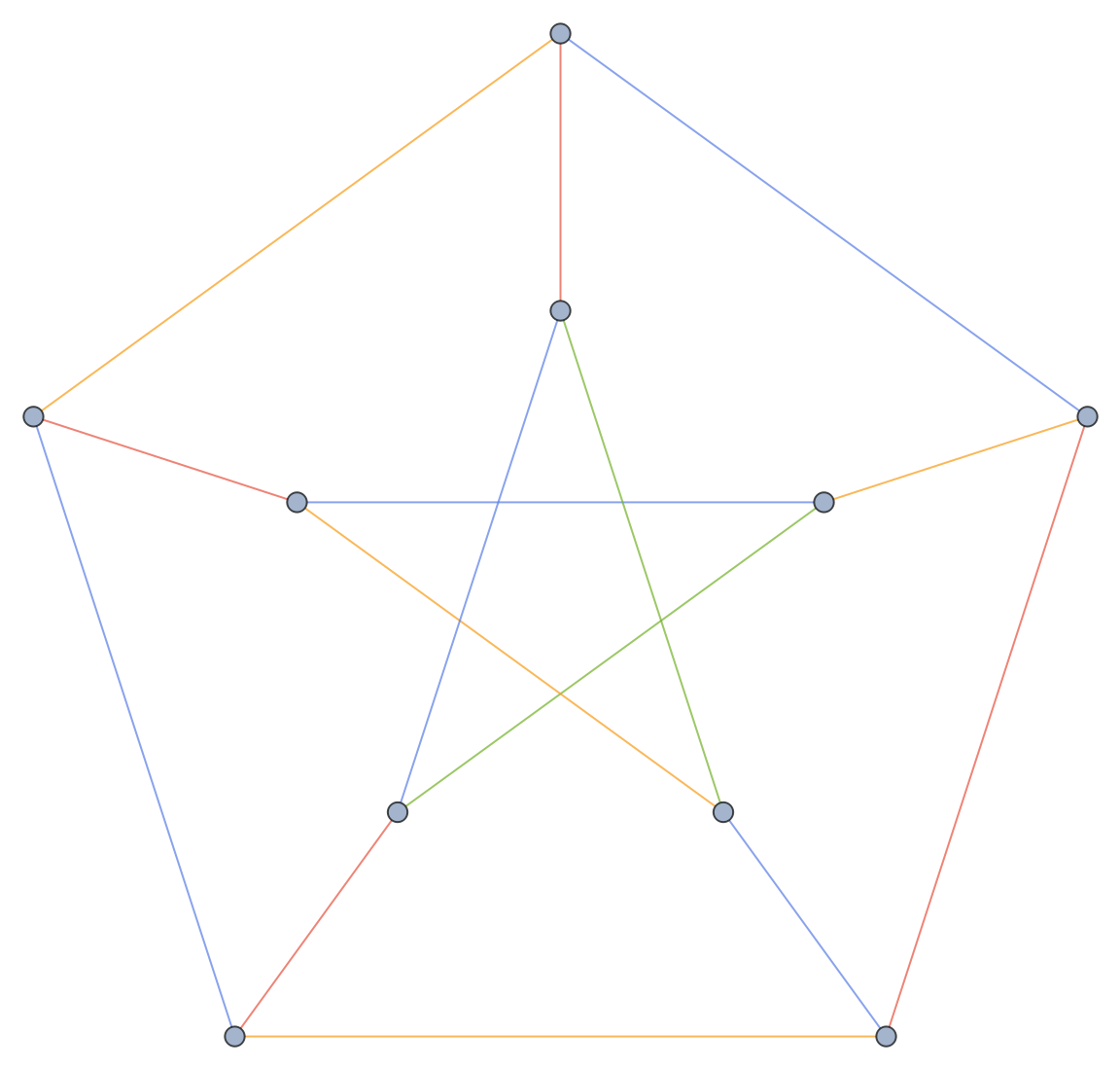### Applications (2)

Find edge colorings for a large random graph:

 In:=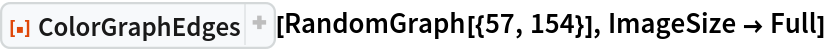Out=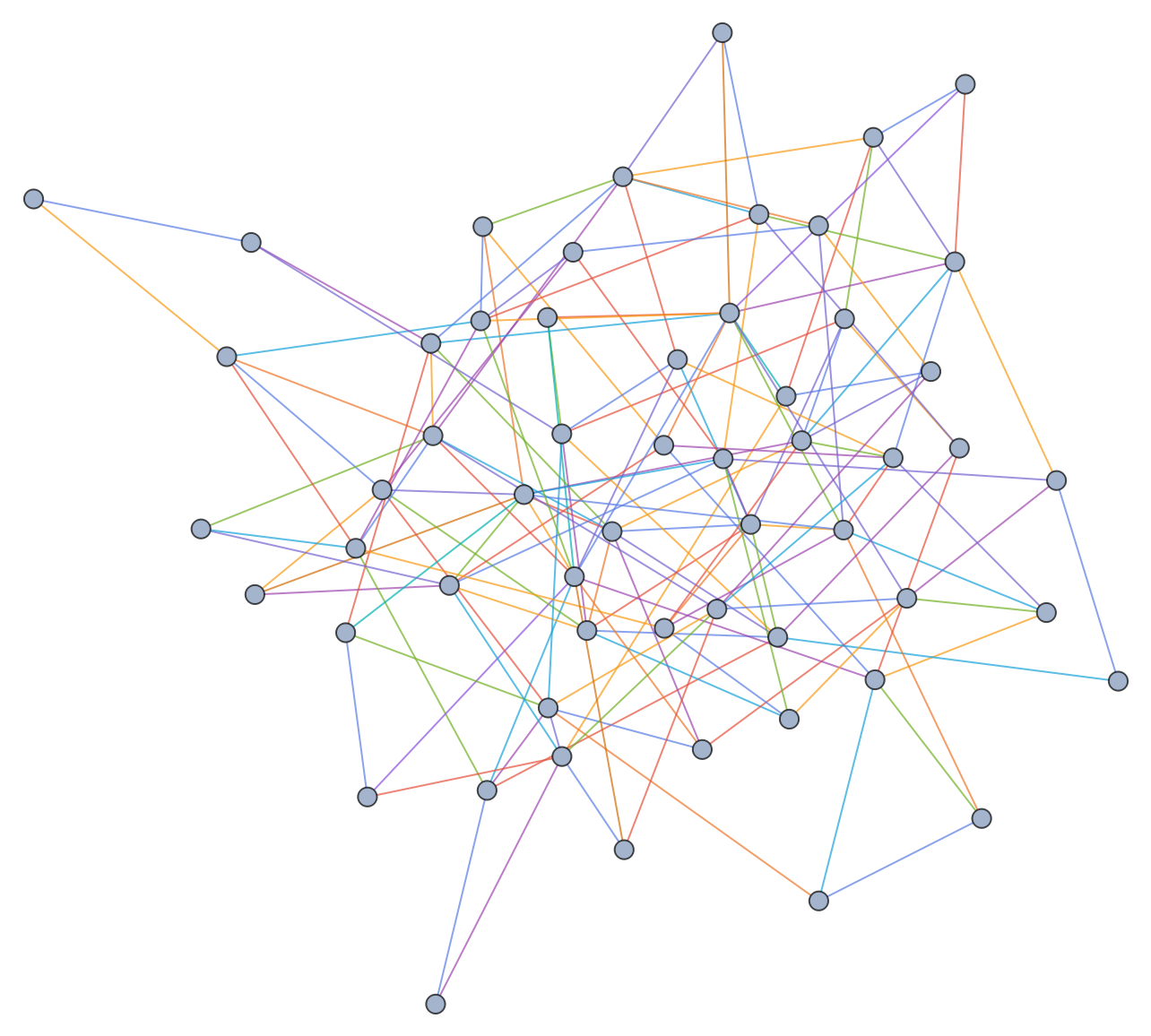Color the Hoffman-Singleton graph with seven colors:

 In:=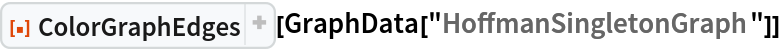Out=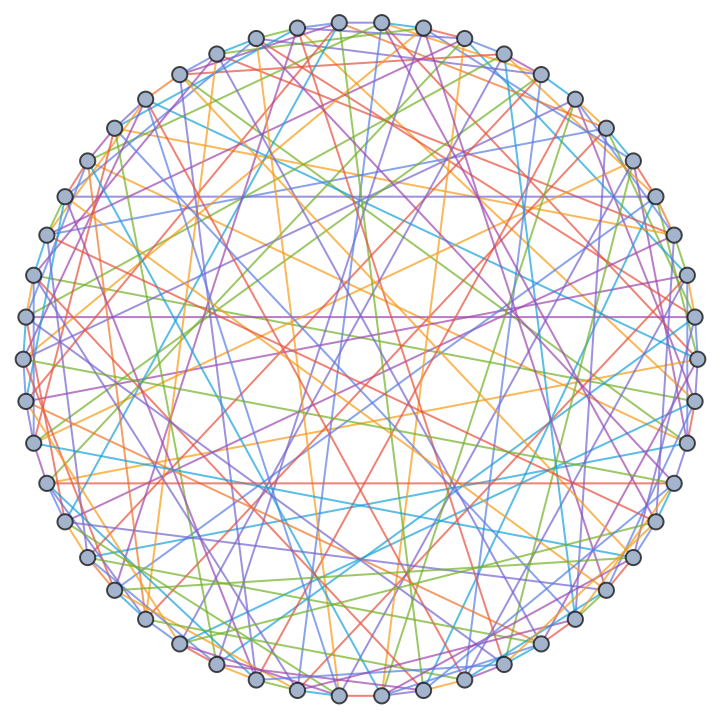### Properties & Relations (2)

Use ColorGraphVertices to color the vertices instead:

 In:=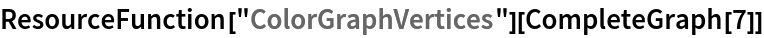Out=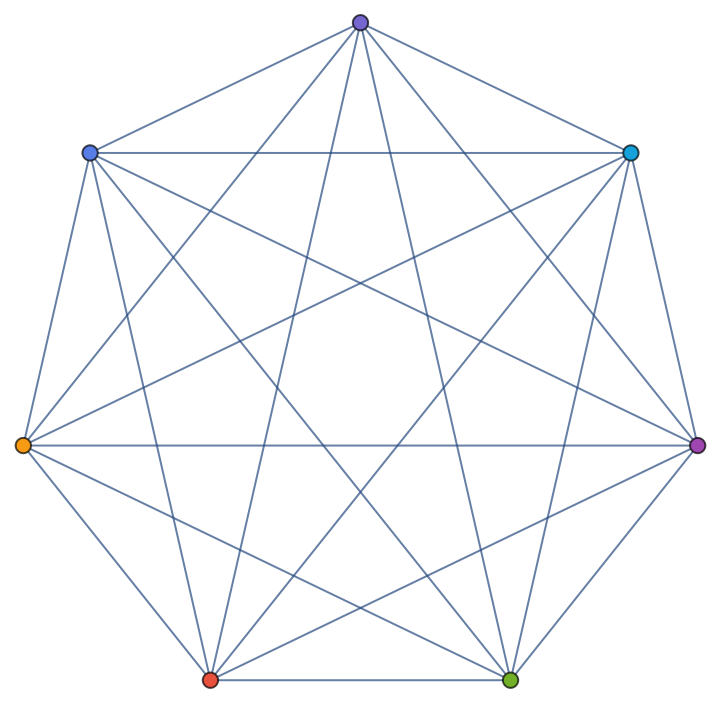This colors the edges:

 In:=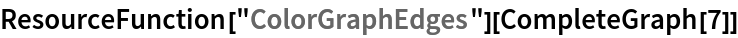Out=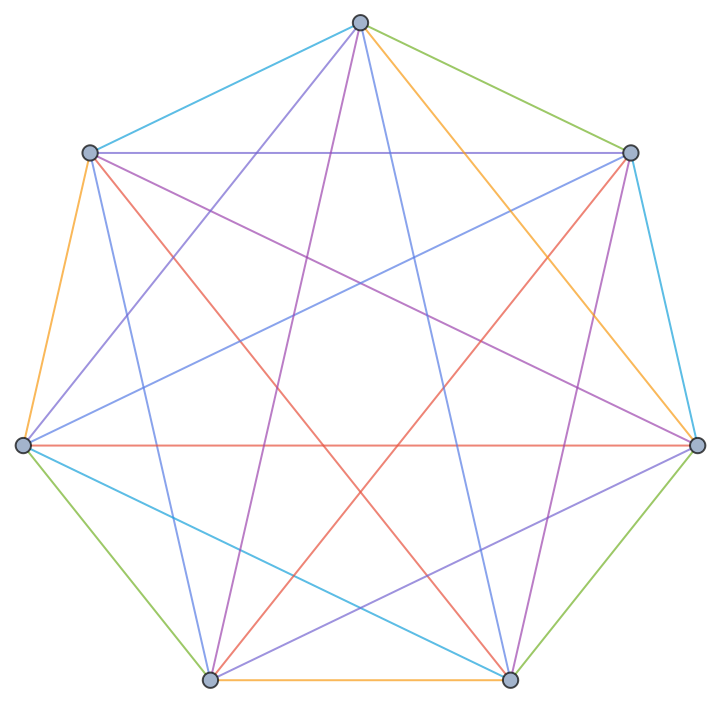The number of colors needed for the edges is given by EdgeChromaticNumber:

 In:=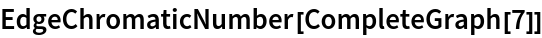Out=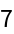Verify the count with FindEdgeColoring:

 In:=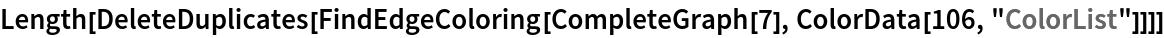Out=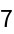### Possible Issues (1)

When the function FindEdgeColoring cannot find an edge coloring, ColorGraphEdges will not color the graph:

 In:=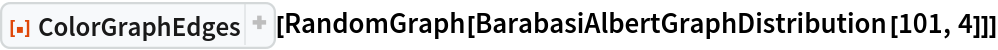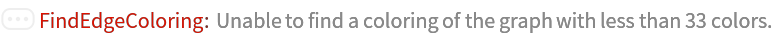Out=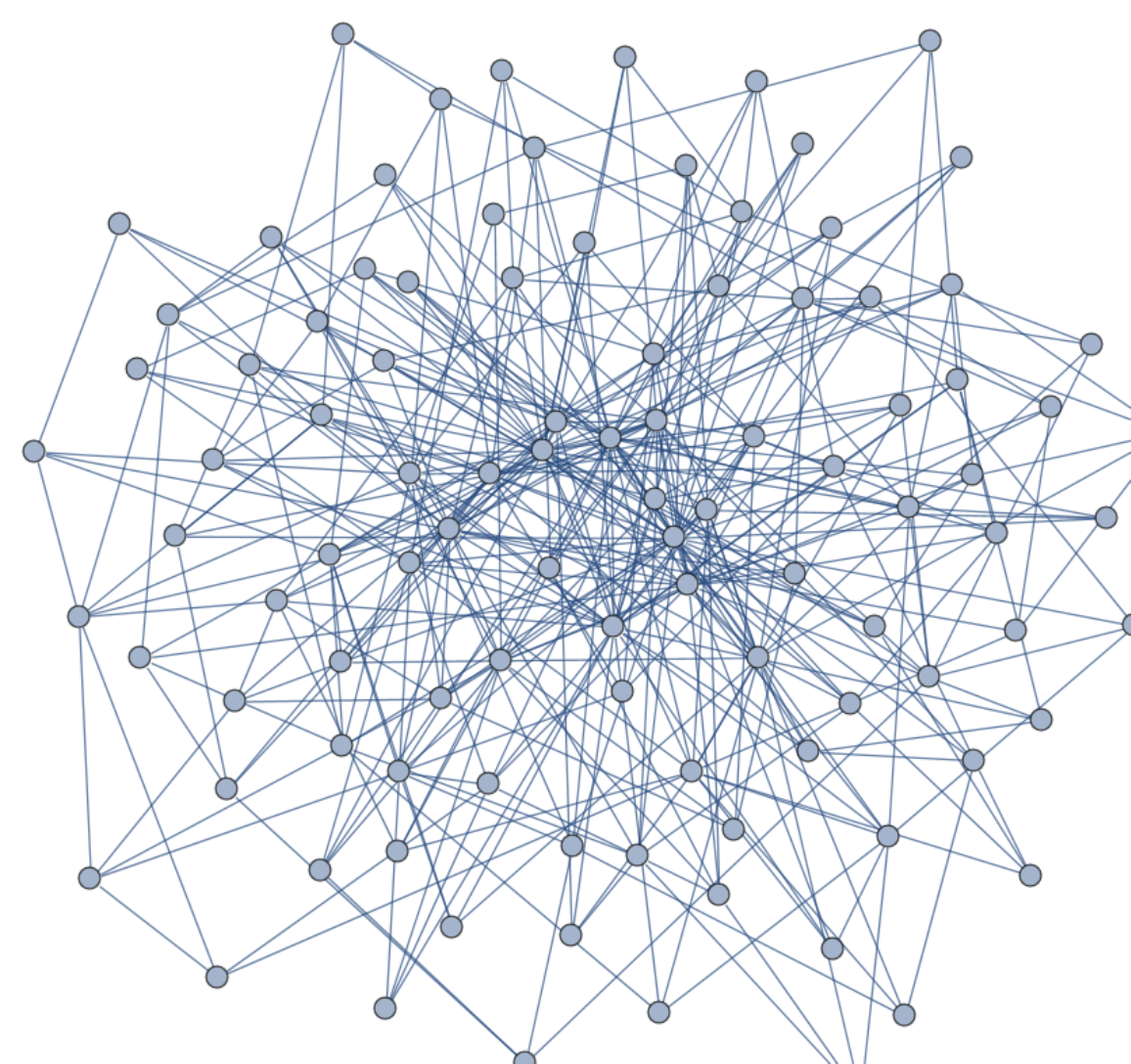Peter Burbery

## Requirements

Wolfram Language 12.3 (May 2021) or above

## Version History

• 1.1.0 – 13 July 2023
• 1.0.0 – 16 August 2022